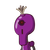# Draw a square of 4cm join the mid point to get another square join the mid points to get a second another join the midpoint at sec

Draw a square of 4cm join the mid point to get another square join the mid points to get a second another join the midpoint at second square to get 3square proceeding like this form a sequence of the square .find the areas , perimeters,diagonals,and lengths of the squares

### 1 thought on “Draw a square of 4cm join the mid point to get another square join the mid points to get a second another join the midpoint at sec”

1.